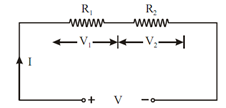## Voltage and Current Division Rules for Combination of Two Resistances Assignment Help

Assignment Help: >> Resistance - Voltage and Current Division Rules for Combination of Two Resistances

Voltage and Current Division Rules for Combination of Two Resistances:

Voltage Division Rule

V  = V . (R1/ R 1 + R2)   , V2 = V. R2/ (R1+ R2)Figure: Series Resistances

Current Division Rule

I1 = I. (R2/ R 1 + R2)      , I2 = I. R1/ (R1+ R2)Figure: Parallel Resistances